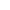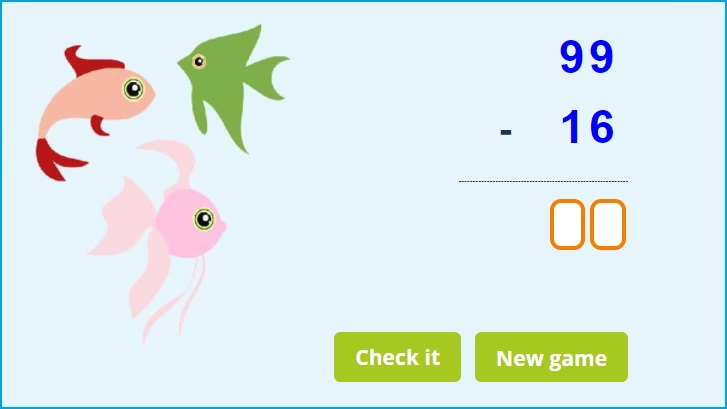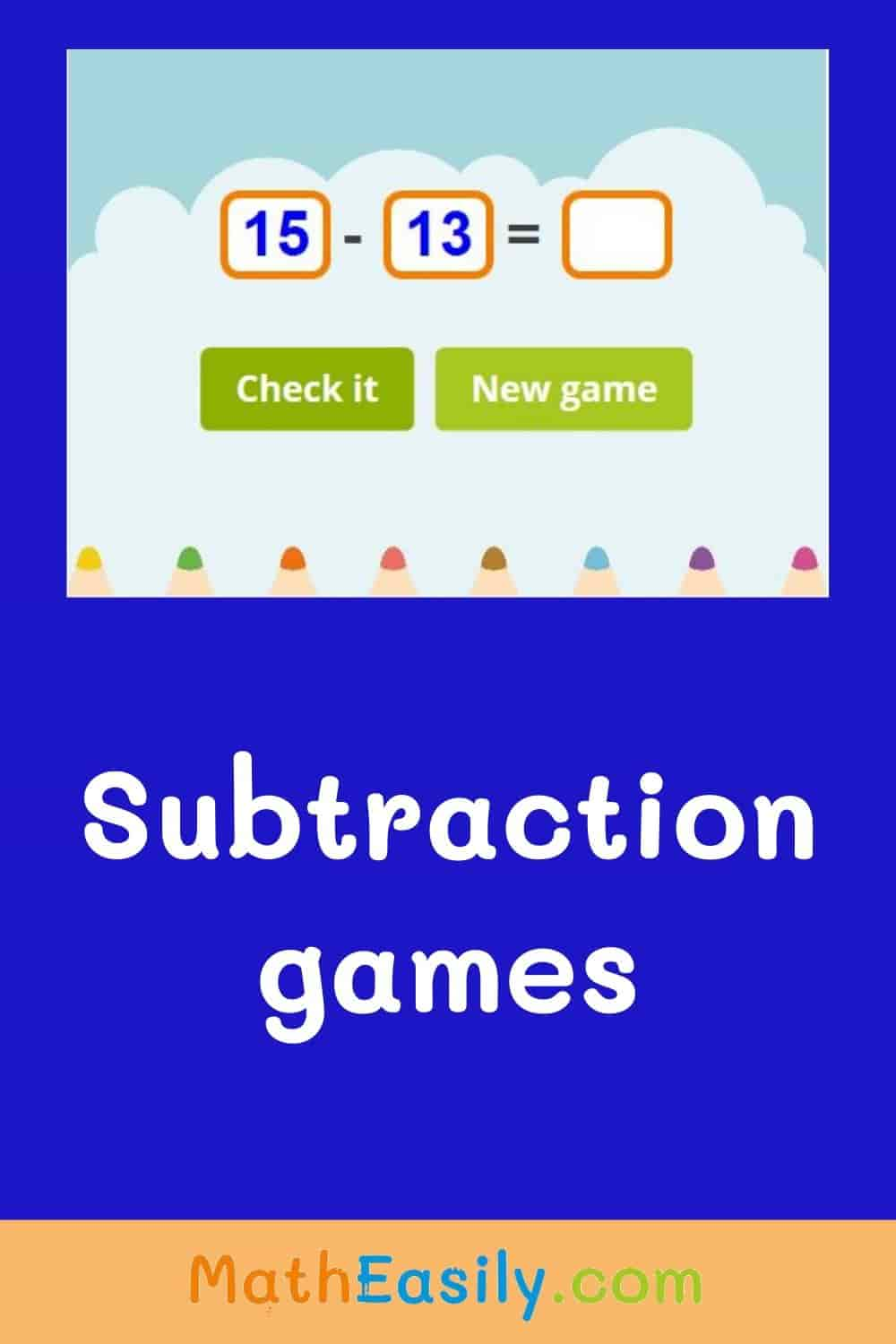﻿ 2 digit subtraction with and without regrouping games

Page No. 1083

# 2 digit subtraction with/without regrouping

2 digit subtraction games. subtraction within 100 worksheets. subtract within 100 with regrouping games for 2nd graders. Double digit subtraction without regrouping online games. 2 digit subtraction without regrouping game. column subtraction year 4. subtract within 100 games. 2nd grade double digit subtraction games online. column subtraction year 3. column subtraction games. Subtract within 100 without regrouping games.

------------------------------------------------------------

New gameSubtract within 100 games: fill in the result of 2 digit subtraction to the empty boxes.
Choose ´Without regrouping´ or ´With regrouping´.

## 2 digit subtraction games onlineInsert the result of two digit subtraction to the empty boxes. Each box should contain only 1 digit. Press "Check it" to find out if your answer is correct. If your result of subtraction is OK, the box will be green. If your answer isn´t correct, the box will be red and you will see the correct number.

Press the button "New game" and play another 2 digit subtraction game:

• ### Subtract within 100 without regrouping games

Click on the blue button No regrouping and play 2 digit subtraction without regrouping games.

• ### Subtract within 100 with regrouping games

Click on the blue button Regrouping and you can play 2 digit subtraction with regrouping games online.

These 2 digit subtraction games teach kids 2 digit subtraction with and without regrouping. You can play subtraction within 100 games online, more than 400 tasks available.

Do you want to practice addition and subtraction at the same time? Then play this addition and subtraction within 100 game.

Do you want to practice basics of subtraction? Then play subtraction pyramid puzzle.

Watch this nice tutorial on YouTube which teaches kids double digit subtraction.

## Free 2 digit subtraction worksheets in PDF

Download free printable two digit subtraction worksheets in PDF. Each worksheet has two pages, the first page is the worksheet and the other page is the subtraction worksheet with answers. You can find even more subtraction worksheets here.## 2 digit subtraction online games

These online ´subtract within 100 games´ help kids to master subtraction within 100.

To play 2 digit subtraction games we assume that kids already have a good knowledge of single digit subtraction within 10 and subtraction within 20.

You can play 2 digit subtraction games online on any device or you can download our free 2 digit subtraction worksheets in PDF.

## How to do two digit subtraction

For example, let’s take the problem: 25 - 17. Starting with the units, you can’t take 7 away from 5.

So, you have to regroup one of the tens in the 20 into a group of ten units. Then, you take away 7 from 15. The answer is 8. Finally, you check the tens side. You have one ten (2-1) minus one ten, which is zero. So, the final answer is 8.

25
- 17
--------
8

## 2 digit subtraction: regrouping games

Children will usually start with 2 digit subtraction without regrouping games and once the kids learn column subtraction (vertical subtraction), they can play 2 digit subtraction with regrouping online games.

Regrouping is a great way to make larger calculations easier to do, we use a column method for it. If kids have mastered 2 digit subtraction then 3 digit subtraction will be a piece of cake for them.

• ### 2 digit subtraction without regrouping games

Subtraction without regrouping means that all digits being subtracted are smaller than their corresponding digits being subtracted from.

In the column method it means than the upper digit is larger or equal than the digit below it. An example would be 35-21 or even 30-20.

• ### 2 digit subtraction with regrouping games

2 digit subtraction with regrouping is a process of exchanging one tens into ten ones. It is used when the minuend is smaller than the subtrahend (in the column). Regrouping is necessary in subtraction when the top number is smaller than the bottom number being subtracted.